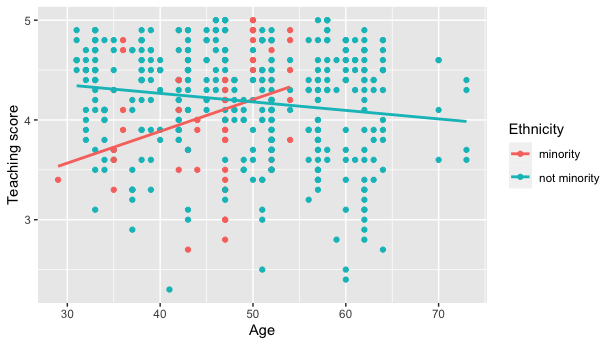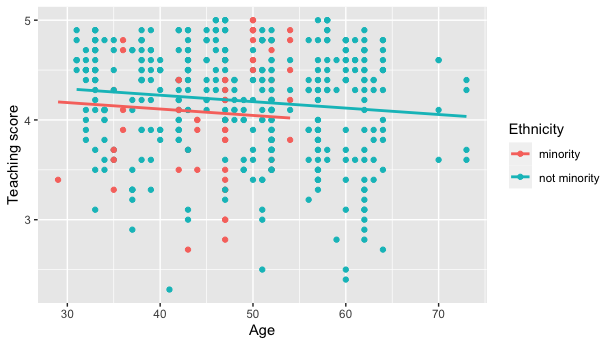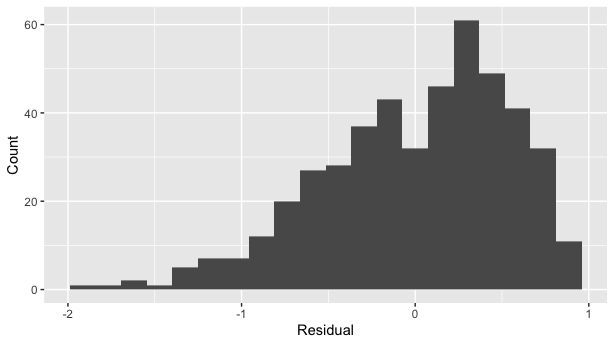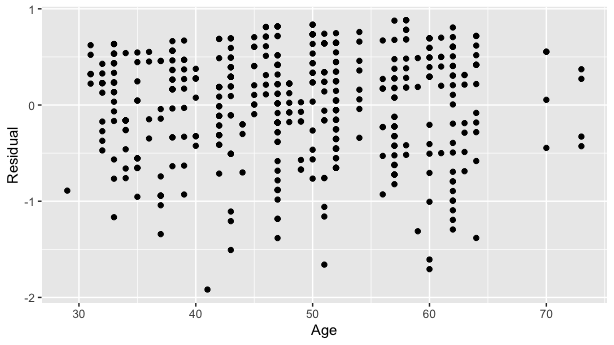# 1 Introduction

Linear regression has long been a staple of introductory statistics courses. While the curricula of introductory statistics courses has much evolved of late, the overall importance of regression remains the same (American Statistical Association Undergraduate Guidelines Workgroup 2016). To facilitate the teaching of regression while leveraging modern computational tools, we’ve created the `moderndive` R package of datasets and functions for tidyverse-friendly introductory linear regression (Wickham, Averick, et al. 2019).

This R package is designed to supplement the book “Statistical Inference via Data Science: A ModernDive into R and the Tidyverse” (Ismay and Kim 2019). Note that the book is also available online at https://www.moderndive.com and is referred to as “ModernDive” for short.

Before we proceed, let’s load all the R packages we are going to need.

``````library(moderndive)
library(ggplot2)
library(dplyr)
library(knitr)
library(broom)``````

Let’s consider data gathered from end of semester student evaluations for a sample of 463 courses taught by 94 professors from the University of Texas at Austin (Diez, Barr, and Çetinkaya-Rundel 2015). This data is included in the `evals` data frame from the `moderndive` package.

In the following table, we present a subset of 9 of the 14 variables included for a random sample of 5 courses1:

1. `ID` uniquely identifies the course whereas `prof_ID` identifies the professor who taught this course. This distinction is important since many professors taught more than one course.
2. `score` is the outcome variable of interest: average professor evaluation score out of 5 as given by the students in this course.
3. The remaining variables are demographic variables describing that course’s instructor, including `bty_avg` (average “beauty” score) for that professor as given by a panel of 6 students.2
ID prof_ID score age bty_avg gender ethnicity language rank
129 23 3.7 62 3.000 male not minority english tenured
109 19 4.7 46 4.333 female not minority english tenured
28 6 4.8 62 5.500 male not minority english tenured
434 88 2.8 62 2.000 male not minority english tenured
330 66 4.0 64 2.333 male not minority english tenured

## 1.1 Regression analysis the “good old-fashioned” way

Let’s fit a simple linear regression model of teaching `score` as a function of instructor `age` using the `lm()` function.

``score_model <- lm(score ~ age, data = evals)``

Let’s now study the output of the fitted model `score_model` “the good old-fashioned way”: using `summary()` which calls `summary.lm()` behind the scenes (we’ll refer to them interchangeably throughout this paper).

``````summary(score_model)
##
## Call:
## lm(formula = score ~ age, data = evals)
##
## Residuals:
##     Min      1Q  Median      3Q     Max
## -1.9185 -0.3531  0.1172  0.4172  0.8825
##
## Coefficients:
##              Estimate Std. Error t value Pr(>|t|)
## (Intercept)  4.461932   0.126778  35.195   <2e-16 ***
## age         -0.005938   0.002569  -2.311   0.0213 *
## ---
## Signif. codes:  0 '***' 0.001 '**' 0.01 '*' 0.05 '.' 0.1 ' ' 1
##
## Residual standard error: 0.5413 on 461 degrees of freedom
## Multiple R-squared:  0.01146,    Adjusted R-squared:  0.009311
## F-statistic: 5.342 on 1 and 461 DF,  p-value: 0.02125``````

Here are 5 common student questions we’ve heard over the years in our introductory statistics courses based on this output:

1. “Wow! Look at those p-value stars! Stars are good, so I should try to get many stars, right?”
2. “How do we extract the values in the regression table?”
3. “Where are the fitted/predicted values and residuals?”
4. “How do I apply this model to a new set of data to make predictions?”
5. “What is all this other stuff at the bottom?”

## 1.2 Regression analysis using `moderndive`

To address these questions, we’ve included three functions in the `moderndive` package that take a fitted model object as input and return the same information as `summary.lm()`, but output them in tidyverse-friendly format (Wickham, Averick, et al. 2019). As we’ll see later, while these three functions are merely wrappers to existing functions in the `broom` package for converting statistical objects into tidy tibbles, we modified them with the introductory statistics student in mind (Robinson and Hayes 2019).

1. Get a tidy regression table with confidence intervals:

``````get_regression_table(score_model)
## # A tibble: 2 x 7
##   term      estimate std_error statistic p_value lower_ci upper_ci
##   <chr>        <dbl>     <dbl>     <dbl>   <dbl>    <dbl>    <dbl>
## 1 intercept    4.46      0.127     35.2    0        4.21     4.71
## 2 age         -0.006     0.003     -2.31   0.021   -0.011   -0.001``````
2. Get information on each point/observation in your regression, including fitted/predicted values and residuals, in a single data frame:

``````get_regression_points(score_model)
## # A tibble: 463 x 5
##       ID score   age score_hat residual
##    <int> <dbl> <int>     <dbl>    <dbl>
##  1     1   4.7    36      4.25    0.452
##  2     2   4.1    36      4.25   -0.148
##  3     3   3.9    36      4.25   -0.348
##  4     4   4.8    36      4.25    0.552
##  5     5   4.6    59      4.11    0.488
##  6     6   4.3    59      4.11    0.188
##  7     7   2.8    59      4.11   -1.31
##  8     8   4.1    51      4.16   -0.059
##  9     9   3.4    51      4.16   -0.759
## 10    10   4.5    40      4.22    0.276
## # … with 453 more rows``````
3. Get scalar summaries of a regression fit including \(R^2\) and \(R^2_{adj}\) but also the (root) mean-squared error:

``````get_regression_summaries(score_model)
## # A tibble: 1 x 9
##   r_squared adj_r_squared   mse  rmse sigma statistic p_value    df
##       <dbl>         <dbl> <dbl> <dbl> <dbl>     <dbl>   <dbl> <dbl>
## 1     0.011         0.009 0.292 0.540 0.541      5.34   0.021     1
## # … with 1 more variable: nobs <dbl>``````

## 1.3 Bonus: Visualizing parallel slopes models with `moderndive`

Furthermore, say you would like to create a visualization of the relationship between two numerical variables and a third categorical variable with \(k\) levels. Let’s create this using a colored scatterplot via the `ggplot2` package for data visualization (Wickham, Chang, et al. 2019). Using `geom_smooth(method = "lm", se = FALSE)` yields a visualization of an interaction model where each of the \(k\) regression lines has their own intercept and slope. For example in , we extend our previous regression model by now mapping the categorical variable `ethnicity` to the `color` aesthetic.

``````# Code to visualize interaction model:
ggplot(evals, aes(x = age, y = score, color = ethnicity)) +
geom_point() +
geom_smooth(method = "lm", se = FALSE) +
labs(x = "Age", y = "Teaching score", color = "Ethnicity")``````Visualization of interaction model.

However, many introductory statistics courses start with the easier to teach “common slope, different intercepts” regression model, also known as the parallel slopes model. However, no argument to plot such models exists within `geom_smooth()`.

Evgeni Chasnovski thus wrote a custom `geom_` extension to `ggplot2` called `geom_parallel_slopes()`; this extension is included in the `moderndive` package. Much like `geom_smooth()` from the `ggplot2` package, you merely add a `geom_parallel_slopes()` layer to the code, resulting in .

``````# Code to visualize parallel slopes model:
ggplot(evals, aes(x = age, y = score, color = ethnicity)) +
geom_point() +
geom_parallel_slopes(se = FALSE) +
labs(x = "Age", y = "Teaching score", color = "Ethnicity")``````Visualization of parallel slopes model.

At this point however, students will inevitably ask a sixth question: “When would you ever use a parallel slopes model?”

## 1.4 Why should you use the `moderndive` package?

To recap this introduction, we believe that the following functions included in the `moderndive` package

1. `get_regression_table()`
2. `get_regression_points()`
3. `get_regression_summaries()`
4. `geom_parallel_slopes()`

are effective pedagogical tools that can help address the six common questions posed by students about introductory linear regression performed in R. We now argue why.

# 2 Features

## 2.1 1. Less p-value stars, more confidence intervals

The first common student question:

“Wow! Look at those p-value stars! Stars are good, so I should try to get many stars, right?”

We argue that the `summary.lm()` output is deficient in an introductory statistics setting because:

1. The `Signif. codes: 0 '' 0.001 '**' 0.01 '*' 0.05 '.' 0.1 ' ' 1` only encourage p-hacking. In case you have not yet been convinced of the perniciousness of p-hacking, perhaps comedian John Oliver can convince you.
2. While not a silver bullet for eliminating misinterpretations of statistical inference, confidence intervals present students with a sense of the associated effect sizes of any explanatory variables. Thus, practical as well as statistical significance is emphasized. These are not included by default in the output of `summary.lm()`.

Instead of `summary()`, let’s use the `get_regression_table()` function:

``````get_regression_table(score_model)
## # A tibble: 2 x 7
##   term      estimate std_error statistic p_value lower_ci upper_ci
##   <chr>        <dbl>     <dbl>     <dbl>   <dbl>    <dbl>    <dbl>
## 1 intercept    4.46      0.127     35.2    0        4.21     4.71
## 2 age         -0.006     0.003     -2.31   0.021   -0.011   -0.001``````

Observe how the p-value stars are omitted and confidence intervals for the point estimates of all regression parameters are included by default. By including them in the output, we can easily emphasize to students that they “surround” the point estimates in the `estimate` column. Note the confidence level is defaulted to 95%.

## 2.2 2. Outputs as tibbles

The second common student question:

“How do we extract the values in the regression table?”

While one might argue that extracting the intercept and slope coefficients can be simply done using `coefficients(score_model)`, what about the standard errors? For example, a Google query of “how do I extract standard errors from lm in R” yielded results from the R mailing list and from Cross Validated suggesting we run:

``````sqrt(diag(vcov(score_model)))
## (Intercept)         age
## 0.126778499 0.002569157``````

We argue that this task shouldn’t be this hard, especially in an introductory statistics setting. To rectify this, the three `get_regression_*` functions all return data frames in the tidyverse-style tibble (tidy table) format (Müller and Wickham 2019). Therefore you can easily extract columns using the `pull()` function from the `dplyr` package (Wickham et al. 2020):

``````get_regression_table(score_model) %>%
pull(std_error)
##  0.127 0.003``````

or equivalently you can use the `\$` sign operator from base R:

``````get_regression_table(score_model)\$std_error
##  0.127 0.003``````

Furthermore, by piping the above `get_regression_table(score_model)` output into the `kable()` function from the `knitr` package (Xie 2020), you can obtain aesthetically pleasing regression tables in R Markdown documents, instead of tables written in jarring computer output font:

``````get_regression_table(score_model) %>%
kable()``````
term estimate std_error statistic p_value lower_ci upper_ci
intercept 4.462 0.127 35.195 0.000 4.213 4.711
age -0.006 0.003 -2.311 0.021 -0.011 -0.001

## 2.3 3. Birds of a feather should flock together: Fitted values & residuals

The third common student question:

“Where are the fitted/predicted values and residuals?”

How can we extract point-by-point information from a regression model, such as the fitted/predicted values and the residuals? (Note we only display the first 10 out of 463 of such values for brevity’s sake.)

``fitted(score_model)``
``````##        1        2        3        4        5        6        7
## 4.248156 4.248156 4.248156 4.248156 4.111577 4.111577 4.111577
##        8        9       10
## 4.159083 4.159083 4.224403``````
``residuals(score_model)``
``````##           1           2           3           4           5
##  0.45184376 -0.14815624 -0.34815624  0.55184376  0.48842294
##           6           7           8           9          10
##  0.18842294 -1.31157706 -0.05908286 -0.75908286  0.27559666``````

But why have the original explanatory/predictor `age` and outcome variable `score` in `evals`, the fitted/predicted values `score_hat`, and `residual` floating around in separate vectors? Since each observation relates to the same course, we argue it makes more sense to organize them together in the same data frame using `get_regression_points()`:

``````score_model_points <- get_regression_points(score_model)
score_model_points``````
``````## # A tibble: 10 x 5
##       ID score   age score_hat residual
##    <int> <dbl> <int>     <dbl>    <dbl>
##  1     1   4.7    36      4.25    0.452
##  2     2   4.1    36      4.25   -0.148
##  3     3   3.9    36      4.25   -0.348
##  4     4   4.8    36      4.25    0.552
##  5     5   4.6    59      4.11    0.488
##  6     6   4.3    59      4.11    0.188
##  7     7   2.8    59      4.11   -1.31
##  8     8   4.1    51      4.16   -0.059
##  9     9   3.4    51      4.16   -0.759
## 10    10   4.5    40      4.22    0.276``````

Observe that the original outcome variable `score` and explanatory/predictor variable `age` are now supplemented with the fitted/predicted values `score_hat` and `residual` columns. By putting the fitted values, predicted values, and residuals next to the original data, we argue that the computation of these values is less opaque. For example, instructors can easily emphasize how all values in the first row of output are computed.

Furthermore, recall that since all outputs in the `moderndive` package are tibble data frames, custom residual analysis plots can be created instead of relying on the default plots yielded by `plot.lm()`. For example, we can check for the normality of residuals using the histogram of residuals shown in .

``````# Code to visualize distribution of residuals:
ggplot(score_model_points, aes(x = residual)) +
geom_histogram(bins = 20) +
labs(x = "Residual", y = "Count")``````Histogram visualizing distribution of residuals.

As another example, we can investigate potential relationships between the residuals and all explanatory/predictor variables and the presence of heteroskedasticity using partial residual plots, like the partial residual plot over age shown in . If the term “heteroskedasticity” is new to you, it corresponds to the variability of one variable being unequal across the range of values of another variable. The presence of heteroskedasticity violates one of the assumptions of inference for linear regression.

``````# Code to visualize partial residual plot over age:
ggplot(score_model_points, aes(x = age, y = residual)) +
geom_point() +
labs(x = "Age", y = "Residual")``````Partial residual residual plot over age.

## 2.4 4. A quick-and-easy Kaggle predictive modeling competition submission!

The fourth common student question:

“How do I apply this model to a new set of data to make predictions?”

With the fields of machine learning and artificial intelligence gaining prominence, the importance of predictive modeling cannot be understated. Therefore, we’ve designed the `get_regression_points()` function to allow for a `newdata` argument to quickly apply a previously fitted model to new observations.

Let’s create an artificial “new” dataset consisting of two instructors of age 39 and 42 and save it in a tibble data frame called `new_prof`. We then set the `newdata` argument to `get_regression_points()` to apply our previously fitted model `score_model` to this new data, where `score_hat` holds the corresponding fitted/predicted values.

``````new_prof <- tibble(age = c(39, 42))
get_regression_points(score_model, newdata = new_prof)
## # A tibble: 2 x 3
##      ID   age score_hat
##   <int> <dbl>     <dbl>
## 1     1    39      4.23
## 2     2    42      4.21``````

Let’s do another example, this time using the Kaggle House Prices: Advanced Regression Techniques practice competition ( displays the homepage for this competition).